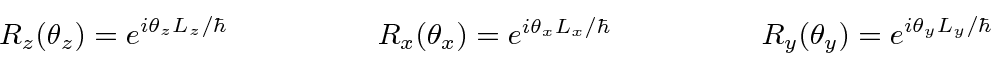## A Study ofOperators and Eigenfunctions

The set of states with the same total angular momentum and the angular momentum operators which act on them are often represented by vectors and matrices. For example the differentstates forwill be represented by a 3 component vector and the angular momentum operators represented by 3X3 matrices. There are both practical and theoretical reasons why this set of states is separated from the states with different total angular momentum quantum numbers. The states are often (nearly) degenerate and therefore should be treated as a group for practical reasons. Also, a rotation of the coordinate axes will not change the total angular momentum quantum number so the rotation operator works within this group of states.

We write our 3 component vectors as follows.The matrices representing the angular momentum operators forare as follows.The same matrices also represent spin 1,, but of course would act on a different vector space.

The rotation operators (symmetry operators) are given byfor the differential form or the matrix form of the operators. Forthese are 3X3 (unitary) matrices. We use them when we need to redefine the direction of our coordinate axes. Rotations of the angular momentum states are not the same as rotations of vectors in 3 space. The components of the vectors represent different quantities and hence transform quite differently. The vectors'' we are using for angular momentum actually should be called spinors when we refer to their properties under rotations and Lorentz boosts.

Jim Branson 2013-04-22